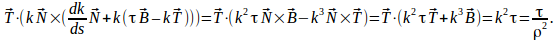## Identity for Torsion and Radius of Curvature

Theorem

For a curve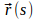where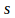is the distance along the curve, if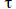is the torsion and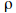is the radius of curvature, then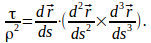Proof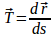(1) and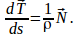Hence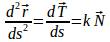(2) where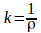is the curvature. Differentiating this expression gives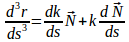One of the Frenet formulae states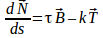where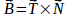is the binormal vector.

Substituting this expression intogives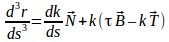(3)

Substitute (1), (2) and (3) into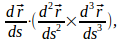obtaining